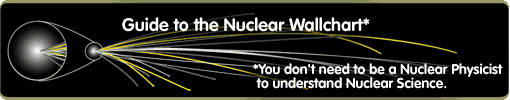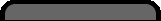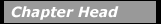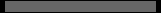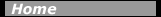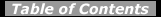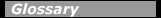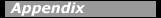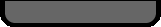## Symmetry in RotationConsider for example the simple idea that when an object is rotated through an angle of 360° it should end in a state no different from its initial state. If we apply this simple symmetry in quantum mechanics, the physics theory of matter and energy at the smallest distances, we find that it imposes the requirement that the angular momentum of rotating objects must be quantized in units of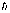(Planck’s constant, h, divided by 2p). A spinning object, be it a planet, a top, or a nucleus, should only be able to have rotations such that its angular momentum comes out in "chunks"in size.

Imagine, then the big surprise that swept through the world of physics when it was discovered in the 1930s that this symmetry was "broken" by particles like electrons, protons and neutrons, which were found to have "spin 1/2", or one half anunit as their "built-in" angular momentum (called "intrinsic angular momentum" or simply "spin"). In nuclear science it has become standard to useas the measuring stick for angular momentum and to describe the angular momentum of nuclei in units of. Thus, we say that a nucleus has angular momentum 0, or 2, or 7/2, in units of.

One consequence of the half-integer spins of neutrons and protons is that nuclei with an odd number of nucleons must have half-integer angular momentum, while nuclei having an even number of nucleons must have integer angular momentum (inunits). Another consequence is quite bizarre: objects with half-integer spin must be rotated by 720° (not 360°) before they return to their initial state! This peculiar behavior has been demonstrated using very slow (ultracold) spin-oriented neutrons from a reactor, which are split into two beams. In one beam the neutrons are rotated about an axis along their direction of motion through some angle, and then the beams are recombined. It is found that when the rotation angle is 360°, the combined beams are out of phase and cancel, (meaning that they are shifted away from the detector) while after 720° of rotation the beams are in phase and reinforced (meaning that they show a large signal at the detector). A rotation of 720° is needed to put the neutrons back in their original state.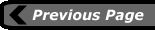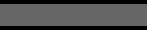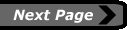last updated: August 9, 2000 webmaster